# Hexagons - polygons with 6 sides

By Martin McBride, 2022-10-10
Tags: hexagon 6 sided shape regular polygon irregular polygon
Categories: gcse geometryA hexagon is a flat shape with 6 straight sides.

## Regular and irregular hexagons

A regular hexagon is a 6-sided shape where every side is the same length and every corner has the same angle. All regular hexagons have the same shape, like this:An irregular hexagon is a 6 sides shape where not every side and angle are equal. There are many different irregular hexagon shapes, here is an example: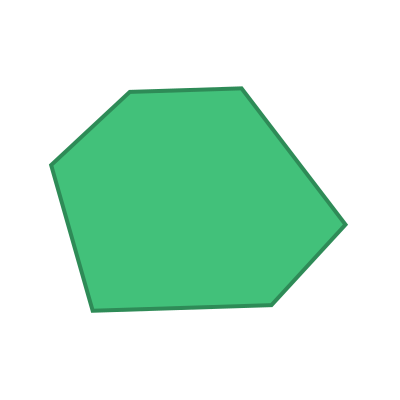## Name

The name hexagon is a combination of the words hex (Greek meaning six) and gonia (Greek meaning corner).

Hexagons are sometimes called 6-gons.

## Interior angles

The interior angles of a hexagon are shown here: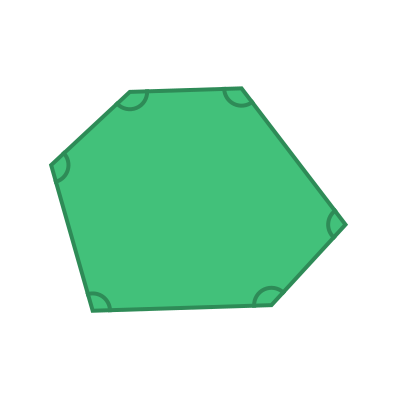The sum of these 6 angles is given by the formula:

sum of interior angles = (n - 2) x 180


Where n is the number of sides. In this case, the number of sides n is 6, so the sum of the interior angles is:

(6 - 2) x 180 = 720 degrees


For a regular hexagon, all the interior angles are equal: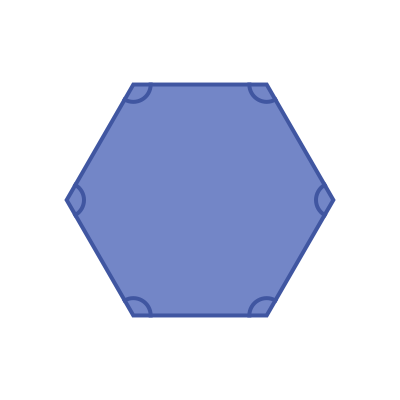This means that the interior angle of a regular hexagon is:

720 / 6 =  120 degrees


## Exterior angles

The exterior angles of a hexagon are shown here: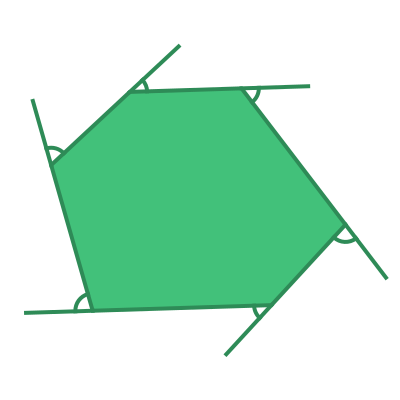The sum of the exterior angles of any polygon is 360 degrees.

For a regular hexagon, all the interior angles are equal: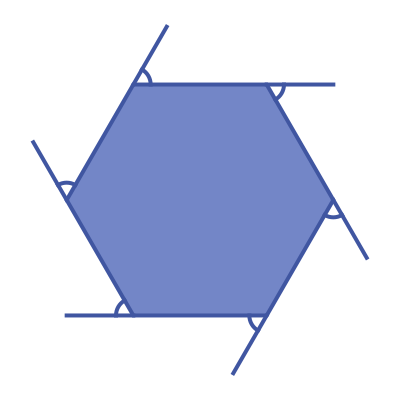This means that the exterior angle of a regular hexagon is:

360 / 6 =  60 degrees


## Symmetry of regular hexagons

A regular hexagon has 6 lines of symmetry. This means that it can be reflected over each of the 6 grey lines shown here: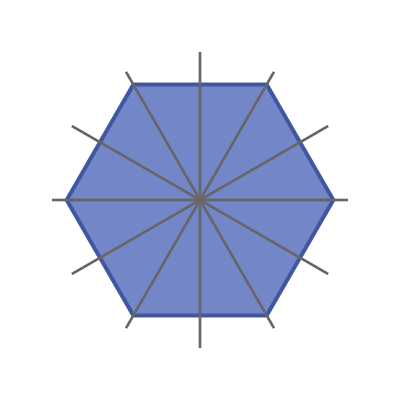A regular hexagon has rotational symmetry of order 6. This means that if it rotated about its centre by a 6th of a full turn, it will map onto itself: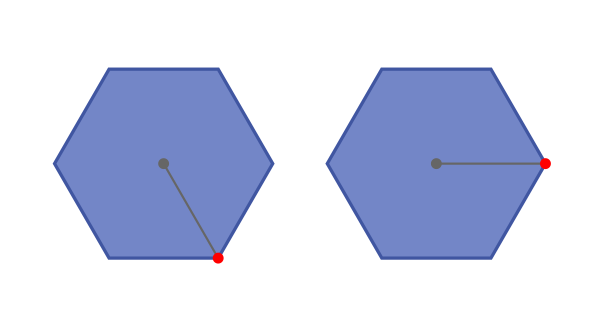## Real life examples

Regular hexagons tessellate, so it is a popular shape for floor tiles. The only other regular polygons that tessellate are squares and equilateral triangles.